## Summary and Guide to Projections

Use projections to display latitude-longitude coordinate data on maps. Choose a projection method by considering these criteria:

These tables show the map projections you can use with map projection structures and map axes. For more information about map projection structures, see `defaultm`. For more information about map axes, see `axesm`.

Note

Most projection IDs are also functions on the MATLAB® search path. These functions are only used in the implementation of functions such as `defaultm` and `axesm`, and therefore their syntaxes are not documented.

### Cylindrical Projections

Projection Name

Projection ID

Equal-AreaConformalEquidistantSpecial Features

Example

Balthasart

`balthsrt`

x

x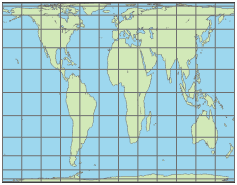Behrmann

`behrmann`

x

x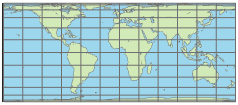Bolshoi Sovietskii Atlas Mira

`bsam`

x

x

x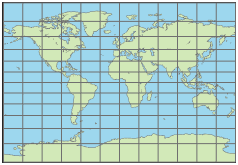Braun Perspective

`braun`

x

x

x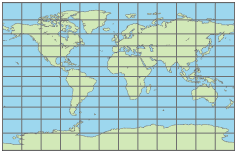Cassini

`cassini`

x

x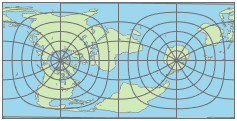Cassini – Standard

`cassinistd`

x

x

x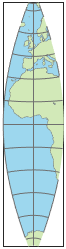Central

`ccylin`

x

x

x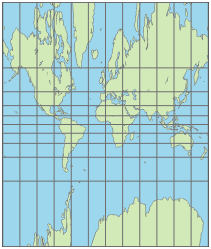Equal-Area Cylindrical

`eqacylin`

x

x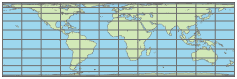Equidistant Cylindrical

`eqdcylin`

x

xGall Isographic

`giso`

x

xGall Orthographic

`gortho`

x

xGall Stereographic

`gstereo`

x

x

x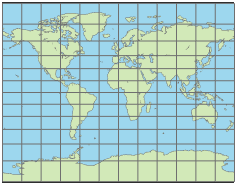Lambert Equal-Area Cylindrical

`lambcyln`

x

xMercator

`mercator`

x

x

Rhumb lines are straight.Miller

`miller`

x

x

xPlate Carrée

`pcarree`

x

x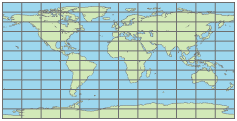Transverse Mercator

`tranmerc`

x

x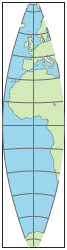Trystan Edwards

`trystan`

x

xUniversal Transverse Mercator (UTM)

`utm`

x

x

Wetch

`wetch`

x

x

x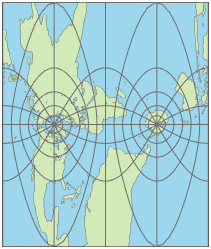### Pseudocylindrical Projections

Projection Name

Projection ID

Equal-Area

Conformal

Equidistant

Special Features

Example

Apianus II

`apianus`

x

x

xCollignon

`collig`

x

x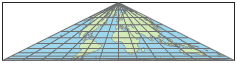Craster Parabolic

`craster`

x

xEckert I

`eckert1`

x

x

x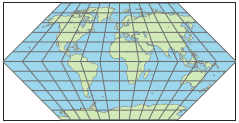Eckert II

`eckert2`

x

xEckert III

`eckert3`

x

x

x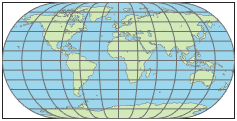Eckert IV

`eckert4`

x

xEckert V

`eckert5`

x

x

x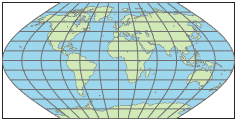Eckert VI

`eckert6`

x

xFournier

`fournier`

x

xGoode Homolosine

`goode`

x

x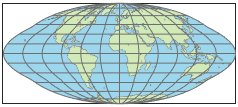Hatano Asymmetrical Equal-Area

`hatano`

x

xKavraisky V

`kavrsky5`

x

xKavraisky VI

`kavrsky6`

x

x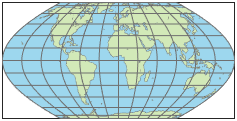Loximuthal

`loximuth`

x

x

x

Rhumb lines from the central point are straight, true to scale, and correct in azimuth.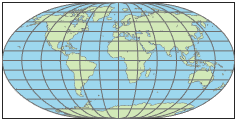McBryde-Thomas Flat-Polar Parabolic

`flatplrp`

x

x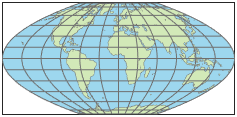McBryde-Thomas Flat-Polar Quartic

`flatplrq`

x

x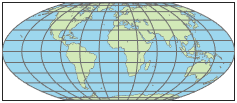McBryde-Thomas Flat-Polar Sinusoidal

`flatplrs`

x

x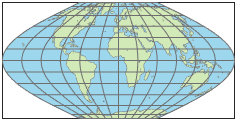Mollweide

`mollweid`

x

xPutnins P5

`putnins5`

x

x

x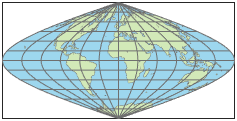Quartic Authalic

`quartic`

x

x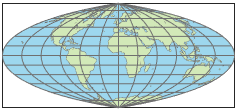Robinson

`robinson`

x

x

xSinusoidal

`sinusoid`

x

x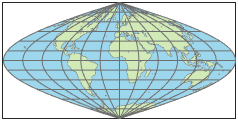Tissot Modified Sinusoidal

`modsine`

x

x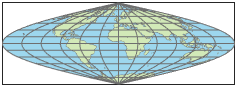Wagner IV

`wagner4`

x

x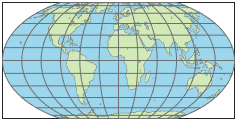Winkel 1

`winkel`

x

x

x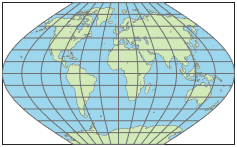### Conic Projections

Projection Name

Projection ID

Equal-Area

Conformal

Equidistant

Special Features

Example

Albers Equal-Area Conic

`eqaconic`

x

xAlbers Equal-Area Conic – Standard

`eqaconicstd`

x

x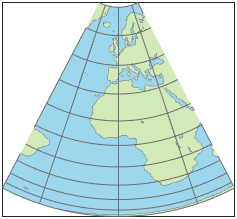Equidistant Conic

`eqdconic`

x

x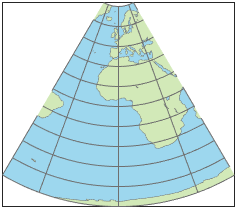Equidistant Conic – Standard

`eqdconicstd`

x

xLambert Conformal Conic

`lambert`

x

xLambert Conformal Conic – Standard

`lambertstd`

x

x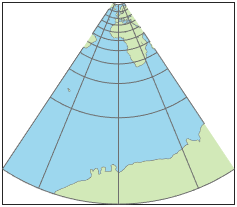Murdoch I Conic

`murdoch1`

x

x

The total area is correct.Murdoch III Minimum Error Conic

`murdoch3`

x

x

The total area is correct.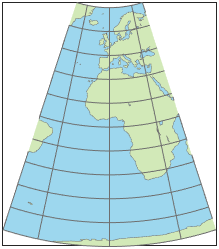### Pseudoconic Projections

Projection Name

Projection ID

Equal-Area

Conformal

Equidistant

Special Features

Example

Bonne

`bonne`

x

x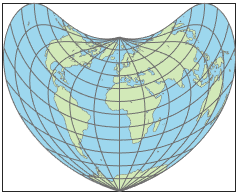Werner

`werner`

x

x### Polyconic Projections

Projection Name

Projection ID

Equal-Area

Conformal

Equidistant

Special Features

Example

Polyconic

`polycon`

x

x

x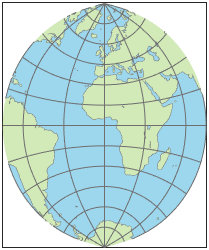Polyconic – Standard

`polyconstd`

x

x

x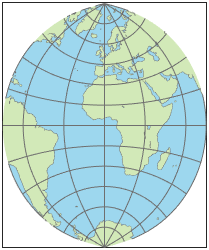Van Der Grinten I

`vgrint1`

x

x

x### Azimuthal Projections

Projection Name

Projection ID

Equal-Area

Conformal

Equidistant

Special Features

Example

Breusing Harmonic Mean

`breusing`

x

x

xEquidistant Azimuthal

`eqdazim`

x

x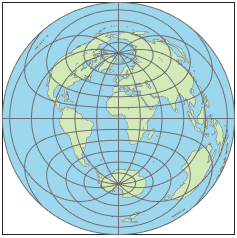Gnomonic

`gnomonic`

x

x

x

Great circles appear as straight lines.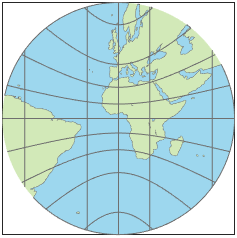Lambert Azimuthal Equal-Area

`eqaazim`

x

x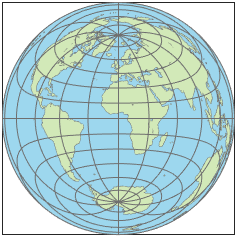Orthographic

`ortho`

x

x

xStereographic

`stereo`

x

x

Great and small circles appear as either straight lines or circular arcs.Universal Polar Stereographic (UPS)

`ups`

x

x

Great and small circles appear as either straight lines or circular arcs.

Vertical Perspective Azimuthal

`vperspec`

x

x

x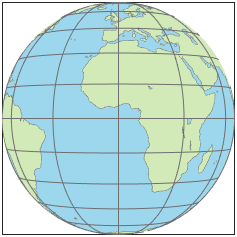### Pseudoazimuthal Projections

Projection Name

Projection ID

Equal-Area

Conformal

Equidistant

Special Features

Example

Wiechel

`wiechel`

x

x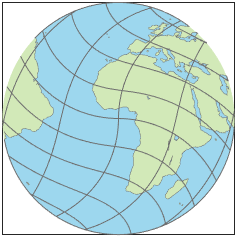### Modified Azimuthal Projections

Projection Name

Projection ID

Equal-Area

Conformal

Equidistant

Special Features

Example

Aitoff

`aitoff`

x

x

x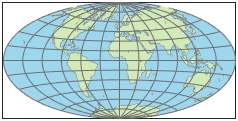Briesemeister

`bries`

x

x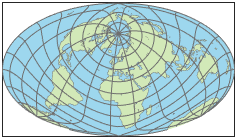Hammer

`hammer`

x

x